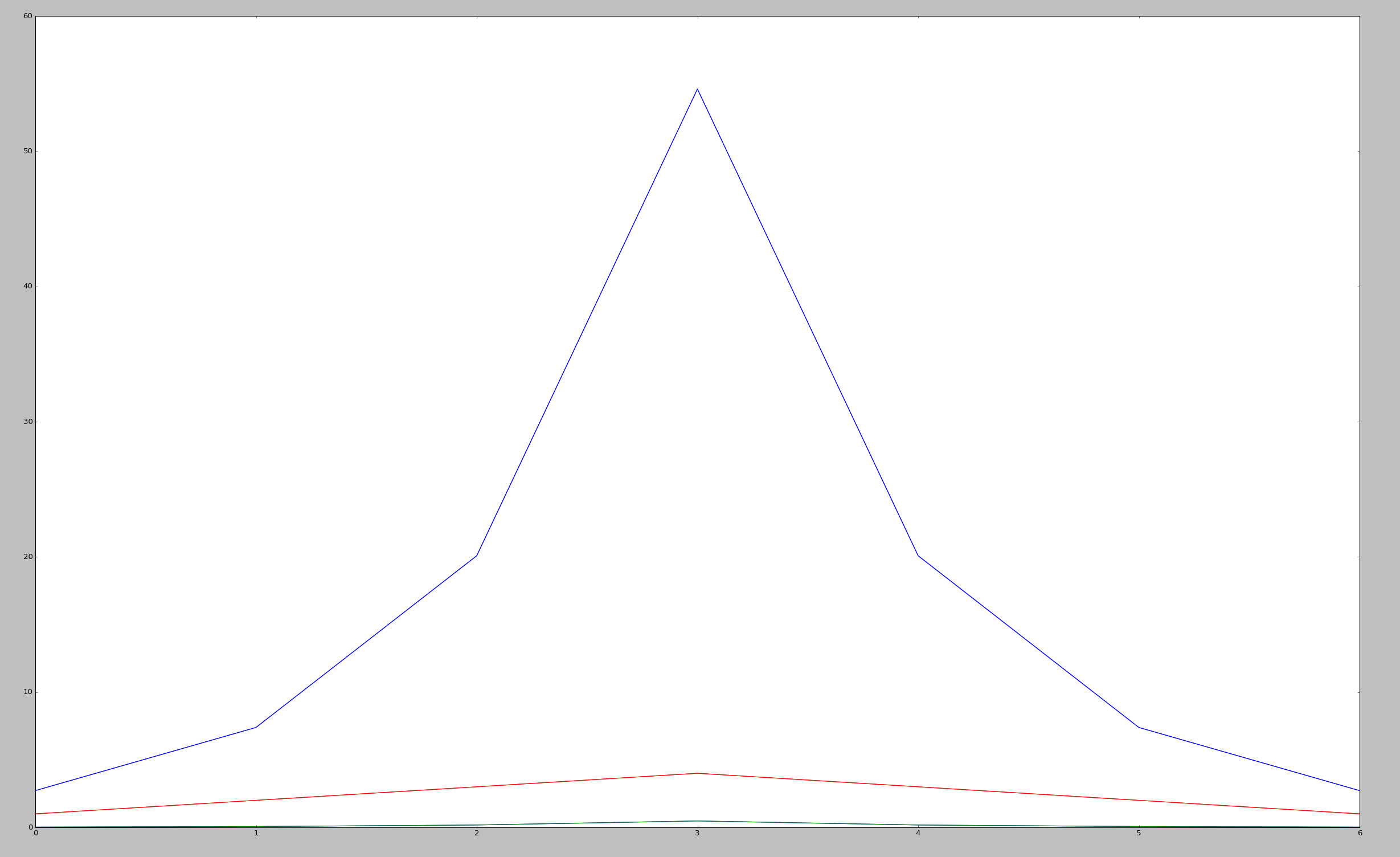Softmax is a function, which intuitively highlights large values and in the same time suppresses values, which are significantly below maximum value. For example, assume we have following sequence of numbers:
[1, 2, 3, 4, 3, 2, 1]

Maximal value is obviously 4.
For every value, we can calculate exponent as

math.exp(i)

By doing this, we get following exponents:

[2.718, 7.389, 20.086, 54.598, 20.086, 7.389, 2.718]

As next, we calculate the sum of all exponet values as
Sum = 2.718 + 7.389 + 20.086 + 54.598 + 20.086 + 7.389 + 2.718 = 114.984

By raising exponents of given values, we exponentialy increase a relative difference between given values.
Finally, we traverse all given values and calculate

softmax(i) = i / Sum

This gives following result, for each value:
[0.024, 0.064, 0.175, 0.475, 0.175, 0.064, 0.024]red: Given values
blue: Exponential values
green:Softmax

This function is often used in Machine Learning algorithms (i.e.: CNTK) for two reasons:

1. To increase a "difference" between values, which is very useful in classification.
2. To normalize calculated "difference" in range 0-1, which will represent a probability in classification.